Next: Existing Partitioning Softwares Up: The State of the Previous: Multilevel approach

### Parallel partitioning algorithms

Although the multilevel approach reduces the computing time significantly, for a very large mesh it can prove to be memory intensive - often exceeding the limits of single CPU memories. Furthermore as the ultimate purpose of partitioning is for the subsequent implementation of the application code on parallel machines, it makes sense to have a parallel partitioning code. Besides, a fast parallel partitioning algorithm can also be used for the purpose of dynamic load balancing. There have been a number of efforts in this area.

Parallel multilevel recursive bisection algorithm PMRSB -

In [2,3], the multilevel spectral bisection was parallelized specifically for the Cray T3D architecture using the Cray SHMEM facility. The linear algebra algorithms (Lanczos, Rayleigh iteration and SYMMLQ) involved are easy to parallelize. Difficulties arose in the parallel graph coarsening, in particular, in the parallel generation of the maximal independent set. These were tackled by using a number of parallel graph theory algorithms from the literature. On a 256 processor Cray T3D, the resulting algorithm PMRSB is able to partition a graph of 1/4 million vertices into 256 subdomains, 140 times faster than a workstation (of similar specification to one processor of the Cray T3D) using an equivalent serial algorithm.

Parallel multilevel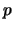-way partitioner ParMETIS -

In , a parallel multilevel-way partitioning scheme was developed. This is based on work in the sequential-way partitioning algorithm METIS , but with some interesting modification to facilitate parallelization. In particular, as in PMRSB [2,3], a parallel algorithm due to Luby  for finding the maximal independent set was employed. However unlike MRSB, where the maximal independent set is used directly to form the coarse graph, here the maximal independent set is used for a different purpose. By considering one independent set at one time, it is possible to avoid conflicts during the coarsening stage when the vertices are matched, as well as during the uncoarsening stage when the boundaries are refined. To illustrate this consider the graph of Figure 11 (a) distributed across two processors. Suppose one hopes to refine the partition to reduce the edge-cut using K-L type algorithms. To do this in parallel without proper scheduling, processor one finds all the vertices that have a positive gain if moved to processor two (those marked with a star), and sends them to processor two; likewise processor two sends all the vertices marked with a circle to processor one. This results in the graph shown in Figure 11 (b) which has the same edge-cut of 13. Any subsequent use of the K-L algorithm in parallel would result in an oscillation between these two graphs. This situation is a result of the fact that moving vertices marked with a star to processor two affects the gains of those marked with a circle, therefore the moves should not be carried out in isolation. One way of avoiding this conflict is for the two processors to communicate with each other and to collaborate on a migration strategy. When there are more than two processors, this may necessitate a coloring of the processor graphs so that processors are grouped in pairs and refinement is carried out on boundaries of the paired processors .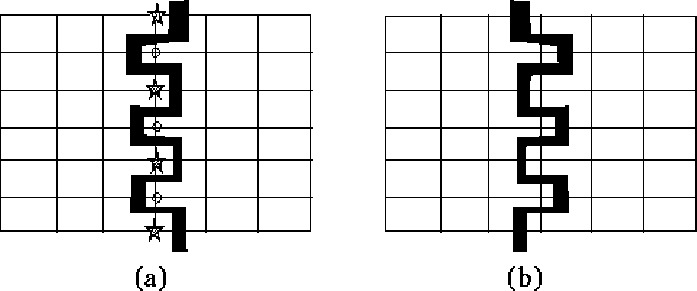An alternative coloring strategy is however used in , as it is believed that pairing the processors lacks the global view of the sequential-way partitioning where each vertex is free to move to the subdomain that leads to the maximum reduction in the edge-cut.

The new strategy is based on coloring the vertices in a number of colors. Vertices of the same color form an independent set. Each colored vertex set is considered one at a time when doing the refinement. By the definition of an independent set (Section 3.4.2), no two vertices in the set are linked by an edge, therefore moving a vertex in this colored independent set to another processor does not affect the gain of other vertices of the same color. Consequently, each processor may decide to send vertices which it identifies as having a positive gain, without the need to communicate with other processors, knowing that such a gain will be realized. This procedure can be repeated on vertices of each color in turn. Using this strategy Figure 12 (a) is colored using two symbols (either with or without a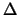). When applying K-L refinement on vertices colored with, Figure 12 (a) becomes Figure 12 (b), which has a smaller edge-cut of 7, instead of the original edge-cut of 13.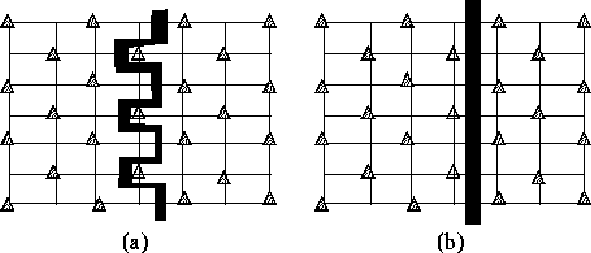The resulting parallel algorithm was implemented on a Cray T3D using its SHMEM communication facility . Table 1  gives a rough idea of the capability of the algorithm and its performance in relation to PMRSB . The two algorithms were applied on two meshes, 598a (a 3D finite element mesh of 144649 nodes and 1074393 edges) and MDUAL (the dual graph of a 3D finite element mesh, with 258569 vertices and 523132 edges). ParMETIS is significantly faster and gives smaller edge-cut. The scalability of both approaches is similar with approximately three to four fold improvement in performance as the number of processor elements (PEs) is increased by a factor of eight.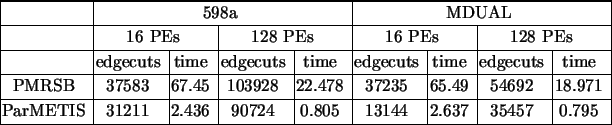A coarse grained modification suitable for MPI implementation was introduced more recently . In this version, the vertex coloring is not used to avoid the need for global point to point communication when each color is considered during the coarsening and refinement phase. Graph folding to part of the parallel processors is also carried at coarse levels to reduce the communication. During the coarsening stage, each processor examines its vertices, and chooses their heavy edge neighbors for matching. A match request of vertex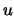with a local neighbor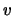is granted immediately while a request for match with a remote neighboris sent to the remote processor for consideration only if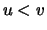. During the refinement phase, conflict is avoided by allowing flow in one direction at a time. That is, a vertexbelongs to partition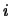is considered for moving to partition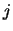only if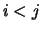(or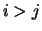at the next round). Applying this strategy to Figure 12 (a), assuming that the flow is from the right to the left, results in Figure 12 (b). Using MPI, this version of the ParMetis algorithm was demonstrated  to lead to much better performance than that based on vertex coloring.

Parallel multilevel-way partitioner JOSTLE-MD -

The parallel partitioning algorithm in  used a different refinement strategy to that of vertex coloring. As this algorithm is designed also for dynamic load balancing, the initial partitioning is assumed to be unbalanced. For any two neighboring subdomainsand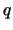, the flow (the amount of load to be migrated to achieve global load balance) is first calculated. The flow fromtois denoted as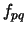. Let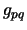denote the total weight of the vertices on the boundary ofwhich have a preference to migrate to. Let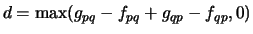, which represents the total weight of all boundary vertices with a positive gain after the flow is satisfied. Then the load to be migrated fromtois given by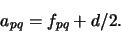(8)

This allows the flow to be satisfied and at the same time an additional load of equal amount is exchanged between the two processors to optimize the edge-cut.

Having decided on the amount of load to be migrated, the idea of relative gain is then used to work out which vertices are to be migrated. The relative gain of a vertexon processoris defined as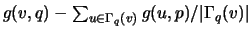, where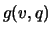denotes the gain of movingto processor, and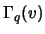those vertices in processorthat are connected with. The vertices on the boundaries are sorted by their relative gain and a weight of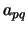is migrated. This avoids the collisions that arise when the gain of vertices varies. But in a situation when each boundary vertex has exactly the same gain, a tie break strategy is necessary. This was not detailed in . The algorithm has been shown to be very efficient in partitioning and re-balancing the meshes resulting from adaptive refinement. The parallel JOSTLE algorithm has been compared with ParMETIS. It was found to give partitioning of better quality, although it runs slower than ParMETIS .

Other parallel graph partitioning algorithms -

In  a parallel single level algorithm, combining inertia bisection with K-L refinement, was implemented. Possible conflict during the refinement stage was avoided by the pairing of processors based on the edge-coloring of the processor graph. The quality of the partition was not as good as multilevel algorithms.

In  a spectral inertia bisection algorithm was introduced. The spectral basis set of eigenvectors for the coarsest graph was first calculated and serves as the spectral coordinates of the vertices. The graph was partitioned with the inertia bisection algorithm (Section 3.1.2), based on these spectral coordinates. Part of the algorithm was parallelized. This algorithm is also suitable for dynamic load balancing, on applications where the mesh is enriched by refining the individual elements. In such a case the refinement can be captured by updating the vertex weights of the dual graph of the mesh, without changing the graph itself. The mesh is repartitioned quickly after refinement, using the spectral information originally calculated for the top level coarse mesh. Since the size of the dual graph does not change, the repartitioning time does not change with the increase of the mesh size, as the refinement steps are carried out. However a possible disadvantage of this approach is that in order to reuse the spectral information, only the vertex weights, not the edge weights, are allowed to be updated. Thus the edge-cut may not be a good representation of the actual edge-cut of the mesh after many levels of refinement.Next: Existing Partitioning Softwares Up: The State of the Previous: Multilevel approach

2000-03-21